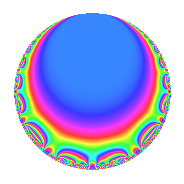# Properties

 Label 33.2.dLevel 33 Weight 2 Character orbit d Rep. character $$\chi_{33}(32,\cdot)$$ Character field $$\Q$$ Dimension 2 Newforms 1 Sturm bound 8 Trace bound 0

# Related objects

## Defining parameters

 Level: $$N$$ = $$33 = 3 \cdot 11$$ Weight: $$k$$ = $$2$$ Character orbit: $$[\chi]$$ = 33.d (of order $$2$$ and degree $$1$$) Character conductor: $$\operatorname{cond}(\chi)$$ = $$33$$ Character field: $$\Q$$ Newforms: $$1$$ Sturm bound: $$8$$ Trace bound: $$0$$

## Dimensions

The following table gives the dimensions of various subspaces of $$M_{2}(33, [\chi])$$.

Total New Old
Modular forms 6 6 0
Cusp forms 2 2 0
Eisenstein series 4 4 0

## Trace form

 $$2q$$ $$\mathstrut +\mathstrut q^{3}$$ $$\mathstrut -\mathstrut 4q^{4}$$ $$\mathstrut -\mathstrut 5q^{9}$$ $$\mathstrut +\mathstrut O(q^{10})$$ $$2q$$ $$\mathstrut +\mathstrut q^{3}$$ $$\mathstrut -\mathstrut 4q^{4}$$ $$\mathstrut -\mathstrut 5q^{9}$$ $$\mathstrut -\mathstrut 2q^{12}$$ $$\mathstrut +\mathstrut 11q^{15}$$ $$\mathstrut +\mathstrut 8q^{16}$$ $$\mathstrut -\mathstrut 12q^{25}$$ $$\mathstrut -\mathstrut 8q^{27}$$ $$\mathstrut +\mathstrut 10q^{31}$$ $$\mathstrut -\mathstrut 11q^{33}$$ $$\mathstrut +\mathstrut 10q^{36}$$ $$\mathstrut -\mathstrut 14q^{37}$$ $$\mathstrut +\mathstrut 11q^{45}$$ $$\mathstrut +\mathstrut 4q^{48}$$ $$\mathstrut +\mathstrut 14q^{49}$$ $$\mathstrut +\mathstrut 22q^{55}$$ $$\mathstrut -\mathstrut 22q^{60}$$ $$\mathstrut -\mathstrut 16q^{64}$$ $$\mathstrut -\mathstrut 26q^{67}$$ $$\mathstrut +\mathstrut 11q^{69}$$ $$\mathstrut -\mathstrut 6q^{75}$$ $$\mathstrut +\mathstrut 7q^{81}$$ $$\mathstrut +\mathstrut 5q^{93}$$ $$\mathstrut +\mathstrut 34q^{97}$$ $$\mathstrut -\mathstrut 11q^{99}$$ $$\mathstrut +\mathstrut O(q^{100})$$

## Decomposition of $$S_{2}^{\mathrm{new}}(33, [\chi])$$ into irreducible Hecke orbits

Label Dim. $$A$$ Field CM Traces $q$-expansion
$$a_2$$ $$a_3$$ $$a_5$$ $$a_7$$
33.2.d.a $$2$$ $$0.264$$ $$\Q(\sqrt{-11})$$ $$\Q(\sqrt{-11})$$ $$0$$ $$1$$ $$0$$ $$0$$ $$q+\beta q^{3}-2q^{4}+(1-2\beta )q^{5}+(-3+\beta )q^{9}+\cdots$$